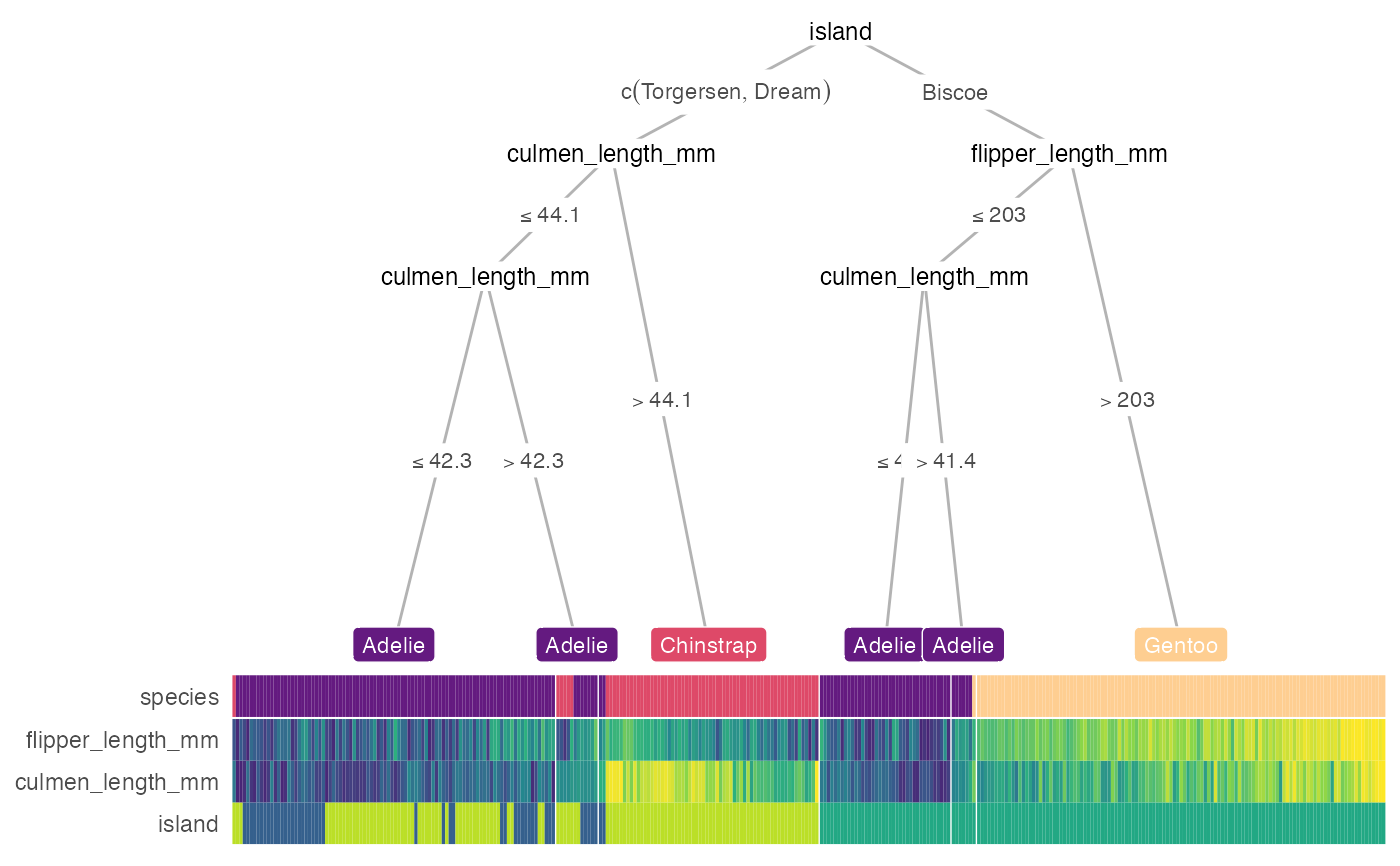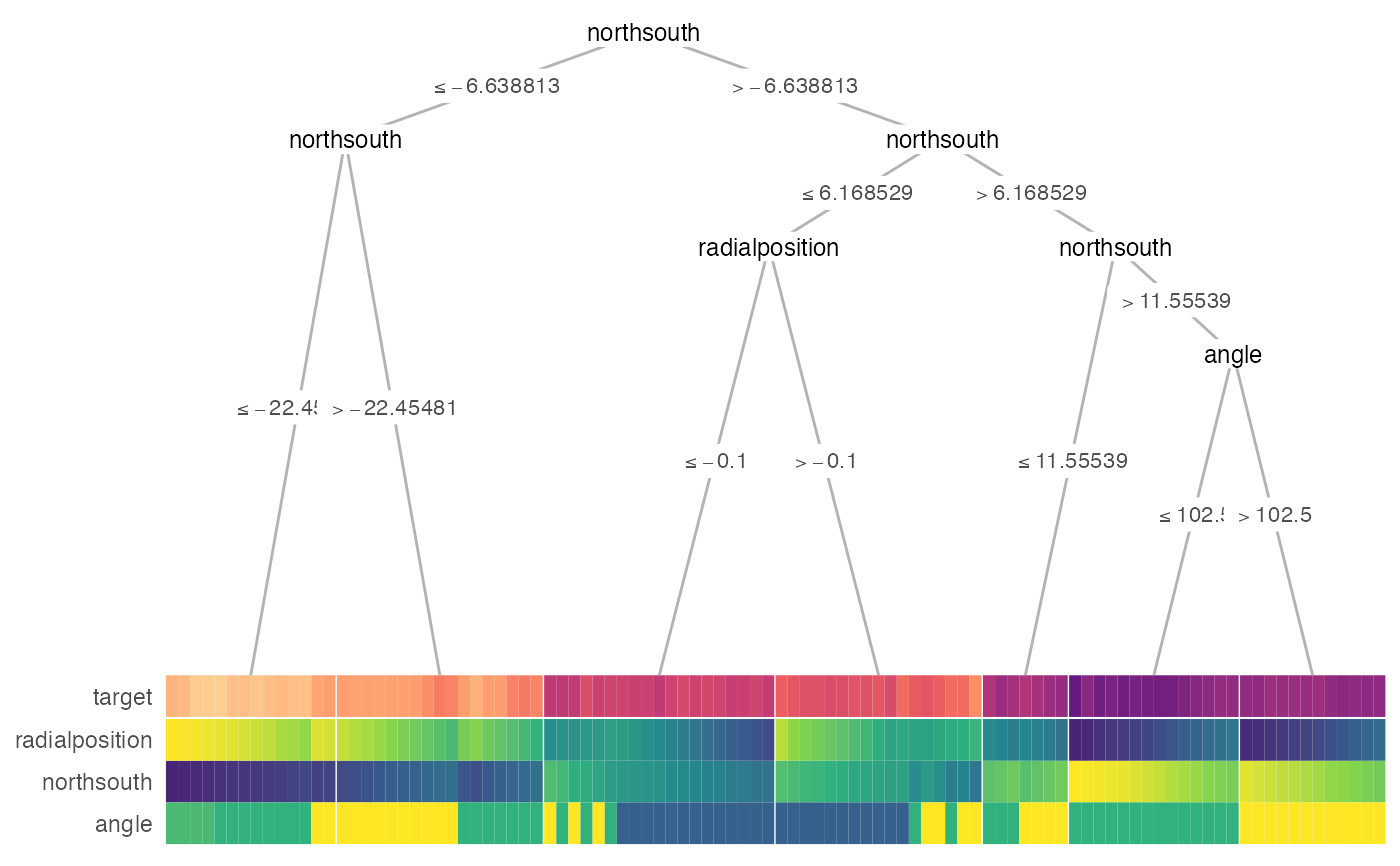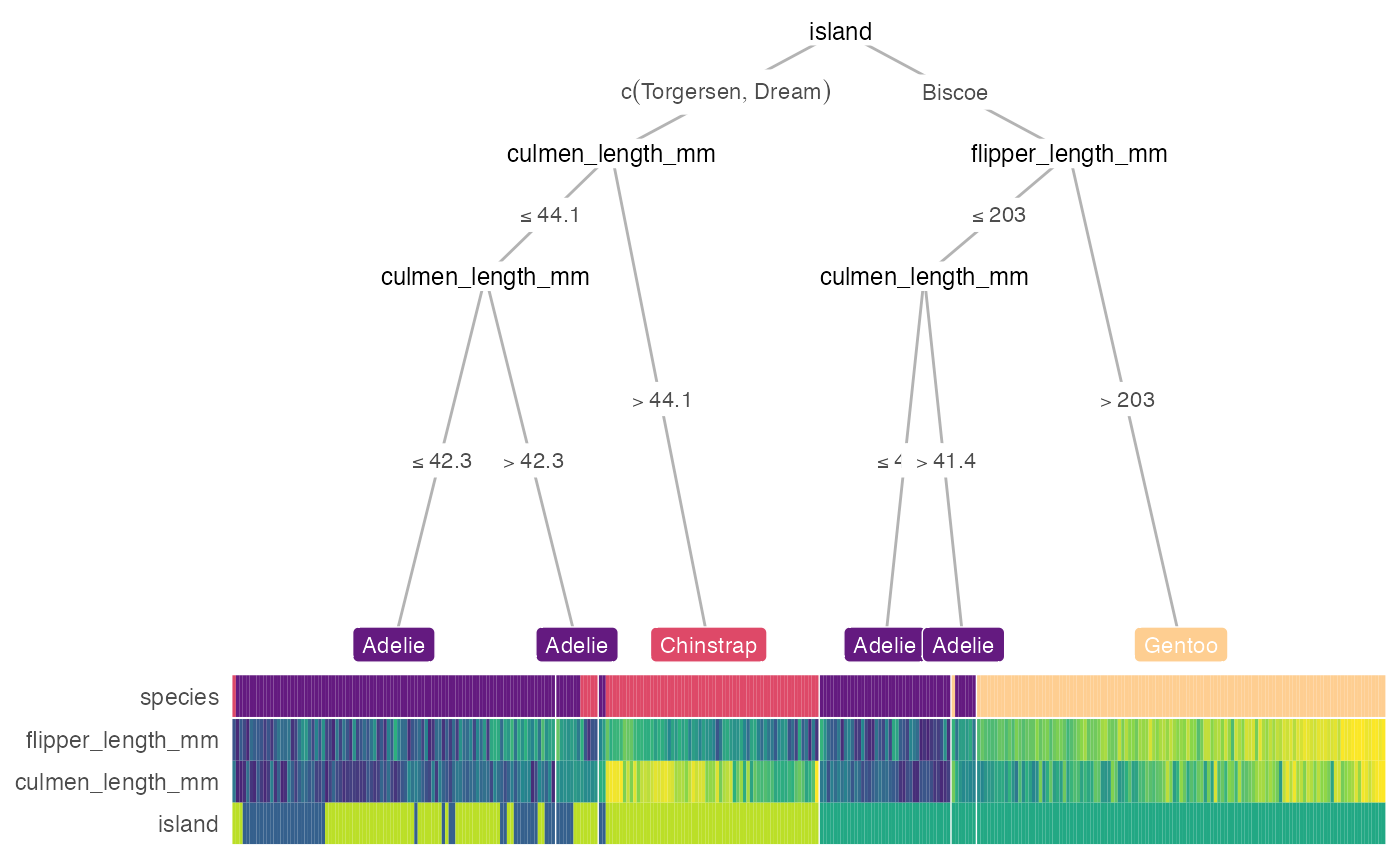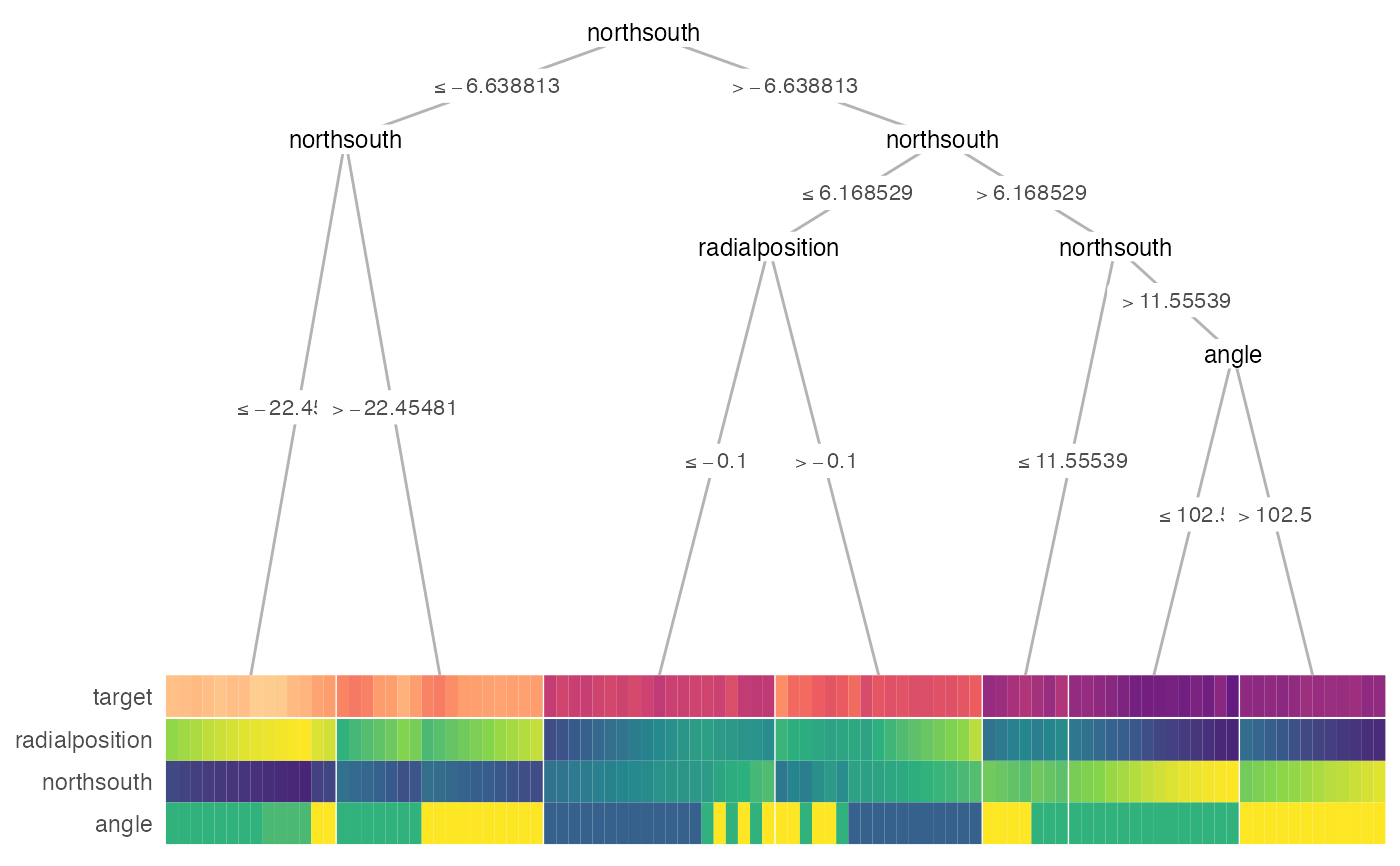heat_tree() alias.

heat_tree(
x,
target_lab = NULL,
data_test = NULL,
feat_types = NULL,
label_map = NULL,
target_cols = NULL,
target_legend = FALSE,
clust_samps = TRUE,
clust_target = TRUE,
custom_layout = NULL,
show = "heat-tree",
heat_rel_height = 0.2,
lev_fac = 1.3,
panel_space = 0.001,
print_eval = (!is.null(data_test)),
...
)

treeheatr(
x,
target_lab = NULL,
data_test = NULL,
feat_types = NULL,
label_map = NULL,
target_cols = NULL,
target_legend = FALSE,
clust_samps = TRUE,
clust_target = TRUE,
custom_layout = NULL,
show = "heat-tree",
heat_rel_height = 0.2,
lev_fac = 1.3,
panel_space = 0.001,
print_eval = (!is.null(data_test)),
...
)

## Arguments

x

Dataframe or a party or partynode object representing a custom tree. If a dataframe is supplied, conditional inference tree is computed. If a custom tree is supplied, it must follow the partykit syntax: https://cran.r-project.org/web/packages/partykit/vignettes/partykit.pdf

target_lab

Name of the column in data that contains target/label information.

data_test

Tidy test dataset. Required if x is a partynode object. If NULL, heatmap displays (training) data x.

Character string indicating the type of problem, either 'classification' (categorical outcome) or 'regression' (continuous outcome).

feat_types

Named vector indicating the type of each features, e.g., c(sex = 'factor', age = 'numeric'). If feature types are not supplied, infer from column type.

label_map

Named vector of the meaning of the target values, e.g., c(0 = 'Edible', 1 = 'Poisonous').

target_cols

Character vectors representing the hex values of different level colors for targets, defaults to viridis option B.

target_legend

Logical. If TRUE, target legend is drawn.

clust_samps

Logical. If TRUE, hierarchical clustering would be performed among samples within each leaf node.

clust_target

Logical. If TRUE, target/label is included in hierarchical clustering of samples within each leaf node and might yield a more interpretable heatmap.

custom_layout

Dataframe with 3 columns: id, x and y for manually input custom layout.

show

Character string indicating which components of the decision tree-heatmap should be drawn. Can be 'heat-tree', 'heat-only' or 'tree-only'.

heat_rel_height

Relative height of heatmap compared to whole figure (with tree).

lev_fac

Relative weight of child node positions according to their levels, commonly ranges from 1 to 1.5. 1 for parent node perfectly in the middle of child nodes.

panel_space

Spacing between facets relative to viewport, recommended to range from 0.001 to 0.01.

print_eval

Logical. If TRUE, print evaluation of the tree performance. Defaults to TRUE when data_test is supplied.

...

Further arguments passed to draw_tree() and/or draw_heat().

## Value

A gtable/grob object of the decision tree (top) and heatmap (bottom).

## Examples

heat_tree(penguins, target_lab = 'species')# \donttest{
heat_tree(
x = galaxy[1:100, ],
target_lab = 'target',
terminal_vars = NULL,
tree_space_bottom = 0)
#> Warning: binary variable(s) 4 treated as interval scaled# }
treeheatr(penguins, target_lab = 'species')treeheatr(
x = galaxy[1:100, ],
target_lab = 'target',